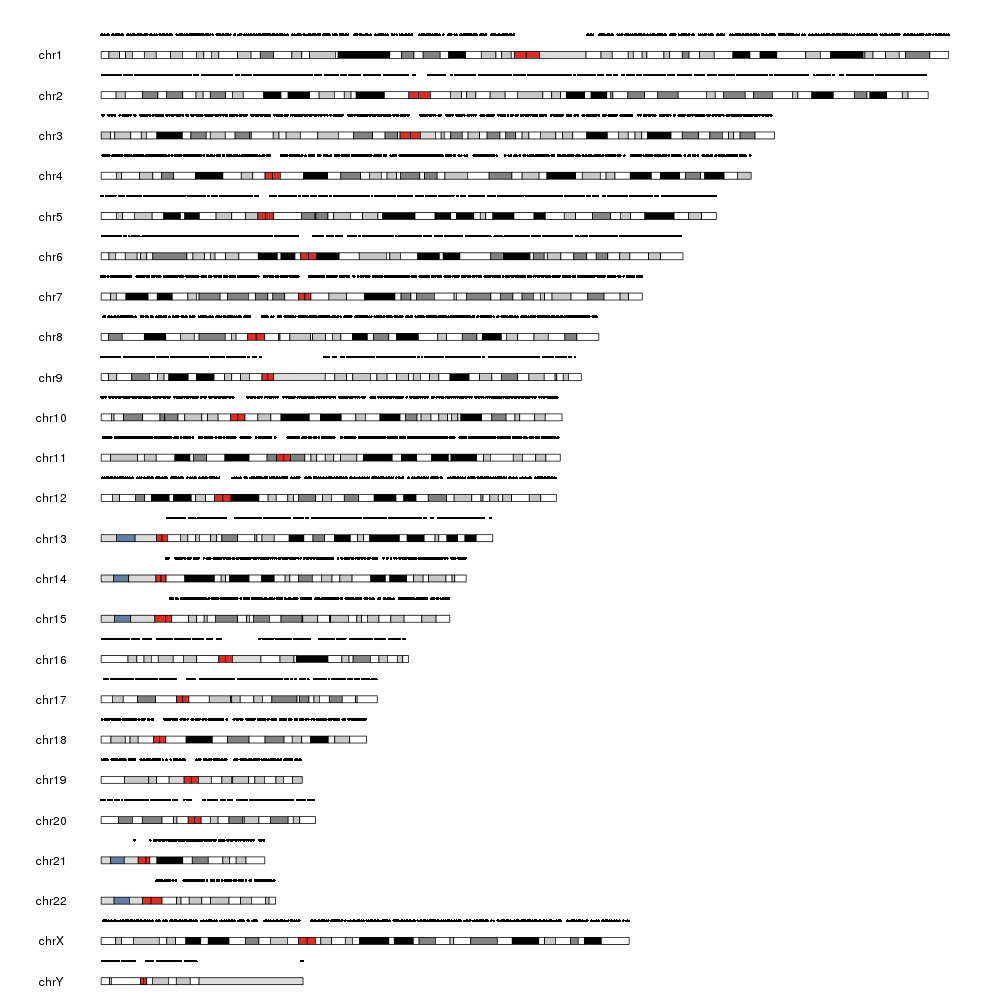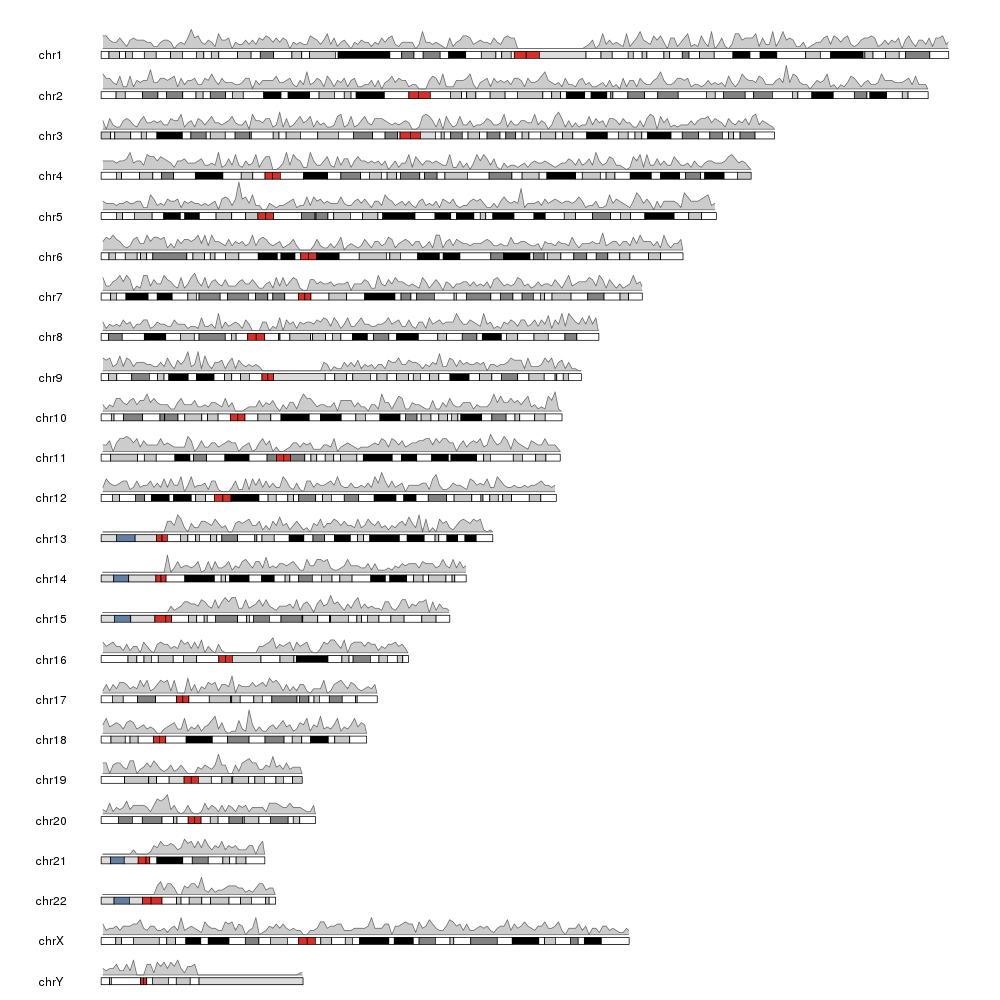Question: snp density plot
0
evelyn110 wrote:

Hello,

I have a `vcf` merge file with multiple samples including chromosome and position of `SNPs`. I want to plot the `SNP` density over separate chromosomes for all samples.

e.g

``````chromosome 1 with SNP density for all samples.
.
.
chromosome n
``````

I found a few posts for plotting with single samples and different file formats. But I want to plot for all samples. A few lines of my file are:

``````#CHROM  POS REF ALT Sample1 Sample2 Sample3
1       635  A   T    T        T      T
1       800  T   A    A        NA     A
1       1234 G   C    C        C      C
``````

Thank you for any suggestions/help!

snp • 1.4k views
written 11 months ago by evelyn110

Hi evelyn,

What do you mean by "SNP density"? in this case all your samples will have data of SNPs in the same positions. Do you mean the density of positions with no NA? or the density of positions with the alternative allele? All these options should be doable with karyoplotetR's kpPlotDensity function but we need to understand exactly what you need.

Hi @bernatgel,

Thank you! This is my first time doing this so I might be confused with the terminology. But I want to plot where the SNPs are located on the chromosomes for all the samples together. I think it will exclude NA's. I also have some heterozygous calls which are converted according to IUPAC codes.

My file is more of a text file because I merged some vcf files and did some analysis including filtering to get a file with a suitable size for downstream analysis. Can this file be used for plotting? Thank you for your help!

2

Hi evelyn,

Ok,let me see if I understand this correctly :) you have information of the same SNPs for all samples, so for all your samples the position and density of the SNPs will be exactly the same.

If you want a single plot with the position (only a few SNPs) or density (if you have many) of the SNPs along the genome ignoring the actual genotype of each sample this can be done with something like this:

``````library(karyoploteR)

#Build a GRanges and convert chromosome names from "1" to "chr1"
vars <- toGRanges(vars[,c(1,2,2,3:length(vars))])
seqlevelsStyle(vars) <- "UCSC"

#Plot SNPs as Points
kp <- plotKaryotype(genome="hg19")
kpPoints(kp, data=vars, y=0.5)

#Plot SNPs as density
kp <- plotKaryotype(genome="hg19")
kpPlotDensity(kp, data=vars)
``````

These images are the results of plotting 10000 random SNPs on the genome with the code above. As you can see if you have more than a few SNPs then plotting the as points do not make much sense.Are these a rough approximation to what you need?

@bernatgel, Thank you! For now, the graphs look like what I need. I tried to use your codes: For this line,

``````vars <- toGRanges(vars[,c(1,2,2,3:length(vars))])
``````

I am getting an error,

``````Error in .Call2("solve_user_SEW0", start, end, width, PACKAGE = "IRanges") :
In range 1: at least two out of 'start', 'end', and 'width', must
be supplied.
1: In toGRanges(vars[, c(1, 2, 2, 3:length(vars))]) :
NAs introduced by coercion
2: In toGRanges(vars[, c(1, 2, 2, 3:length(vars))]) :
NAs introduced by coercion
``````

I am reading the package and command lines but for now I am not able to interpret the error.

Hmm...

The example data you provided is the actual first few lines of your data file? The error is telling you that in some lines of the file POS is not a number. Might it be that there are some NA's in POS? What's the result of

``````anyis.na(vars[,2]))
``````

Yes, my data lines look like what I provided. I just have more samples so I pasted only three samples. I checked the file and POS is number. I ran

``````anyis.na(vars[,2]))
``````

and got

`````` FALSE
``````
1

Oook... that's strange.

What about `all(is.numeric(vars[,2]))`?

Maybe you can try directly

``````vars <- GenomicRanges::GRanges(seqnames=vars[,1], ranges=IRanges::IRanges(start=vars[,2], end=vars[,2))
``````

and see if it works. But somehow, not all your POS are valid numbers for GRanges...

`all(is.numeric(vars[,2]))` gave

`````` TRUE
``````

Thank you, it worked!

Great :)

Glad it worked. I'm concerned that it should have worked with the other code too... would it be possible and feasible to send me the actual data file (or at least a small part (the first 100 lines if it fails with only 100 lines)) to check what's going on? my email is bgel@igtp.cat

Thank you, sure! I want to know one more thing about this plot. I want to add windows/bins of a certain size on each chromosome to give an idea about the size of chromosomes. Is it possible?

1

Does it work for you to add a ruler with the size in megabases?

For that simply use `kpAddBaseNumbers(kp)` (more information in the tutorial)

Yes, my data lines look like what I provided. I just have more samples so I pasted only three samples. I checked the file and POS is number. I ran

``````anyis.na(vars[,2]))
``````

and got

`````` FALSE
``````